# Insertion Sort

The search algorithms in the previous two sections require that the array to be searched is already in sorted order. There are many algorithms for getting an array into this sorted state. This section discusses one of the simplest sorting algorithms, insertion sort.

Insertion sort is the algorithm many people use when sorting a hand of cards. Begin with all of the cards on the table. Pick up one card and place it in the left hand. Pick up a second card and add it to the left hand, either to the left or right of the first card depending on whether it is smaller or larger. Insert a third card into its proper place in this sequence, and so on, until all of the cards have been inserted.

To implement insertion sort on an array of ints, we use an idea from the ArrayList class: we divide the output array into "in use" and "not in use" regions (Figure 8-6). The numbers in the "in use" region are already sorted. When the algorithm finishes, the entire array is in the "in use" region, so all of the numbers are sorted.

Figure 8-6. A first version of insertion sort uses two arrays: one to hold the data still to be inserted (left) and another to hold the result (right). When a number is inserted in the output, any larger numbers have to be shifted over one position.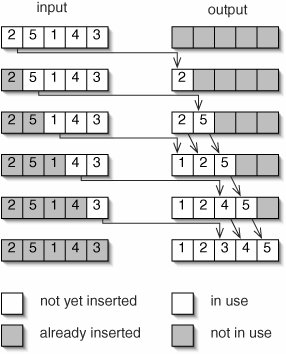The observant reader will notice that the "in use" portion of the input array is always exactly the same size as the "not in use" portion of the output array. We can use the same array for both purposes. In fact, instead of producing a new output array, we can simply rearrange the elements of the input array.

Given this improvement, the first number we actually have to insert is element 1. Element 0 would always be inserted at position 0, and it's already there. It will be moved if any smaller numbers are later inserted. This improved version is illustrated in Figure 8-7.

Figure 8-7. An improved version of insertion sort uses the input array to hold both the sorted portion (unshaded) and the numbers still to be inserted (shaded). In each step, the next available unsorted number is inserted into the sorted region of the array.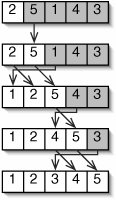The code for insertionSort() is given in Figure 8-8. The main loop inserts each element in turn (except element 0). The loop on lines 68 works through the sorted region from right to left, moving each element one position to the right as it goes. This both finds the correct place for target and makes sure there is room to put it there when it arrives.

Figure 8-8. Code for insertionSort().

 ``` 1 /** Arrange the numbers in data from smallest to largest. */ 2 public static void insertionSort(int[] data) { 3 for (int i = 1; i < data.length; i++) { 4 int target = data[i]; 5 int j; 6 for (j = i - 1; (j >= 0) && (data[j] > target); j--) { 7 data[j + 1] = data[j]; 8 } 9 data[j + 1] = target; 10 } 11 } ```

The running time of insertion sort depends on the running time of this inner loop. This is effectively a linear search through the i numbers which have already been sorted. In the best case, data was already in order, so the inner loop takes a single step for each insertion. The best-case running time for insertion sort is: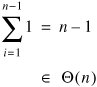In the worst case, data was in reverse order, so each number must be moved all the way to the beginning of the array as it is inserted. The total time required for this is: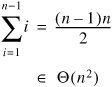In the average case, we can assume that when we insert element i it is equally likely to end up in any of the positions 0 through i. (This is effectively the same assumption we made in analyzing the average-case performance of linear search.) Each insertion is into a sequence of i 1 already sorted numbers, so it takes at least (i 1)/2 comparisons on average. The total average case running time for insertion sort is therefore at least:Since the average-case time can't be worse-than the worst-case time (which is quadratic), the average-case time must also be in O(n2), and therefore in Q(n2).

We will see some more efficient sorting algorithms in Chapters 9, 12, and 14, but we now have a benchmark. Any sorting algorithm that takes time in an order higher than Q(n2) is less efficient than insertion sort and not worth considering.

Remember that asymptotic notation roughly indicates the rate at which a function grows, but obscures constant factors. For example, a method that takes 10n2 milliseconds to run is in a higher order than one that takes 200n milliseconds, but is actually faster for very small values of n. Because it is so simple, insertion sort has a low constant factor within its order. It is therefore a good algorithm to use when the number of elements being sorted is very small.

Exercises

8.6

On line 4 of the code for insertionSort() (Figure 8-8) we store data[i] in a separate variable target. The version in Figure 8-9 omits this "redundant" variable. Explain why it doesn't work.

Figure 8-9. Broken version of insertionSort() for Exercise 8.6.

 ``` 1 /** Arrange the numbers in data from smallest to largest. */ 2 public static void insertionSort(int[] data) { 3 for (int i = 1; i < data.length; i++) { 4 int j; 5 for (j = i - 1; (j >= 0) && (data[j] > data[i]); j--) { 6 data[j + 1] = data[j]; 7 } 8 data[j + 1] = data[i]; 9 } 10 } ```
8.7

What is the amortized running time of insertion sort? (Hint: You should be able to answer this almost instantly, without doing any algebra.)

8.8

Assume that all n! permutations of the elements of data are equally likely (see Exercise 8.1). Argue that, after element i is inserted, it is equally likely to appear in any of the positions 0 through i.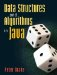Data Structures and Algorithms in Java
ISBN: 0131469142
EAN: 2147483647
Year: 2004
Pages: 216
Authors: Peter Drake